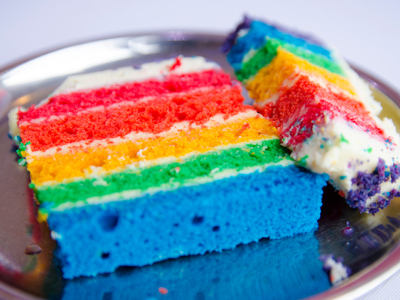The cake's been cut in two but one piece is bigger than the other!

# Year 1 Numbers - Most, Fewest, Least

This quiz addresses the requirements of the National Curriculum KS1 Maths and Numeracy for children aged 5 and 6 in year 1. Specifically this quiz is aimed at the section dealing with recognising most, fewest and least.

Recognising 'most', 'fewest' and 'least' involves looking at numbers or quantities and identifying which has the higher or lower value. This could mean understanding the value of digits within numbers or counting groups of objects to see which have more, most, less, fewest or least.

Question 1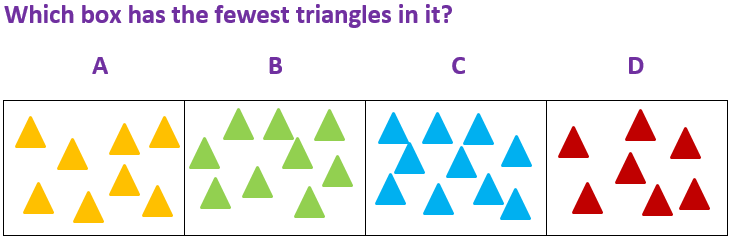A
B
C
D
D has 7 triangles which is the fewest
Question 2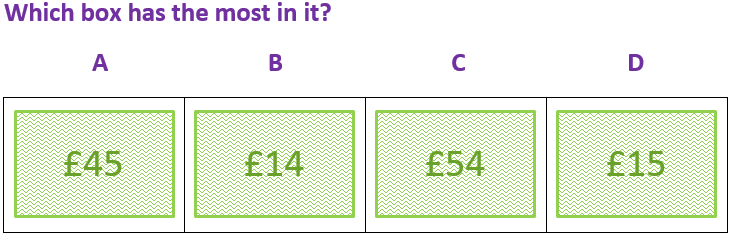A
B
C
D
C has £54 which is the most
Question 3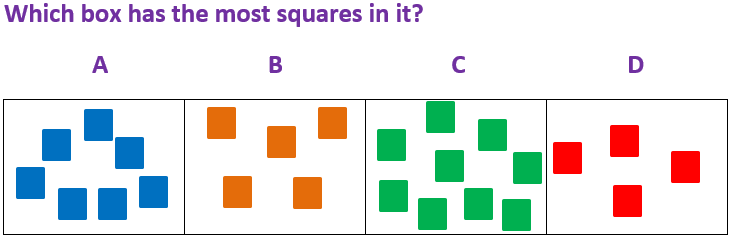A
B
C
D
C has 9 squares which is the most
Question 4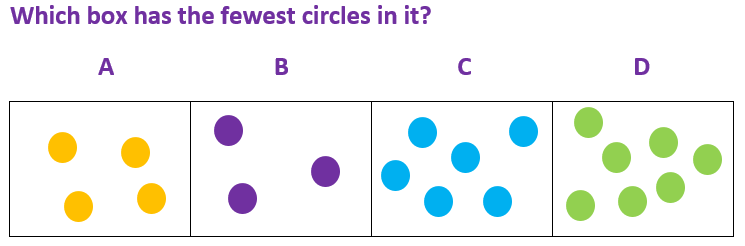A
B
C
D
B has only 3 circles which is the fewest
Question 5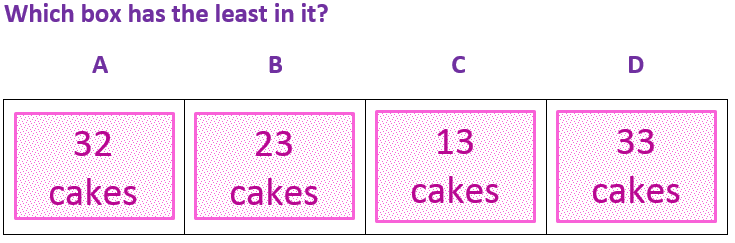A
B
C
D
C has 13 cakes, which is the least
Question 6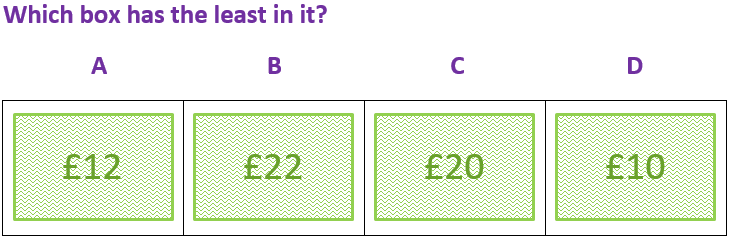A
B
C
D
D has £10, which is the least
Question 7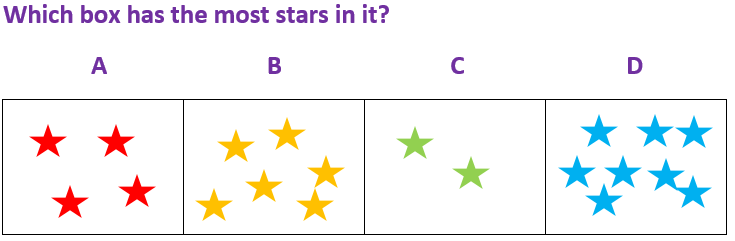A
B
C
D
D has got 8 stars which is the most!
Question 8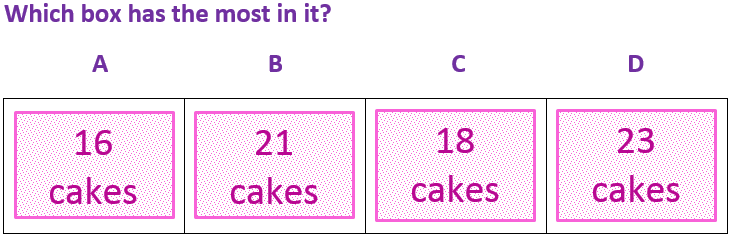A
B
C
D
D has 23 cakes, which is the most. That's a lot of cakes!
Question 9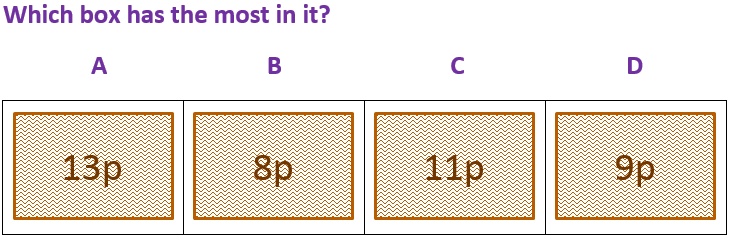A
B
C
D
A has 13p, which is the most
Question 10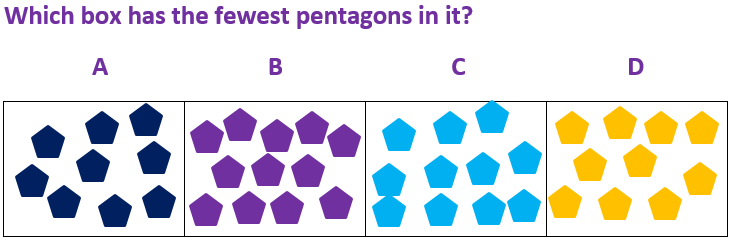A
B
C
D
A has 9 pentagons which is the fewest